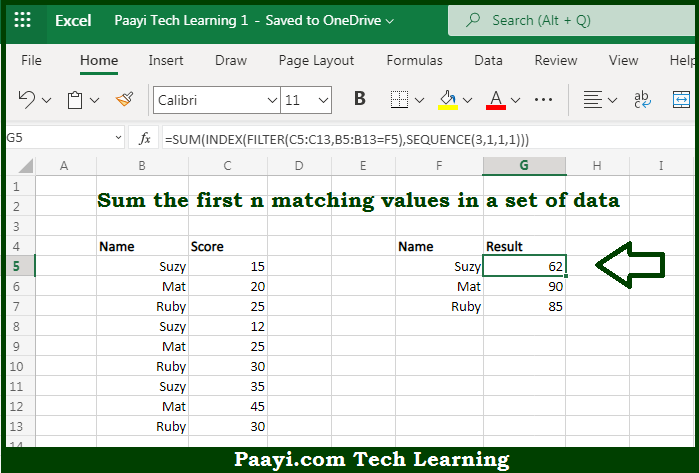# Learn How to SUM First n Matching Values in Microsoft Excel

Written by | 0 Comments | 533 Views

In this article, you will learn how to SUM various things in Microsoft Excel using a single/combination(s) of functions. You will also know how to SUM First n Matching Values and see the generic formula.

SUM First n Matching Values in Microsoft Excel

The main purpose of this formula is to sum the first n matching values in a set of data. Here we will learn how to sum the first n matching values given in the workbook in Microsoft Excel. That implies, with the help of a formula based on the FILTER and SEQUENCE function you can able to sum the first n matching values in a set of data. So, with the help of this formula, you can able to sum the first n matching values given in the workbook in Microsoft Excel.

General Formula to SUM First n Matching Values

=SUM(INDEX(FILTER(range,logic),SEQUENCE(n,1,1,1)))

The Explanation for the SUM First n Matching ValuesSo we know that with the help of the given formula above you can able sum the first n matching values in a set of data. Here we will learn how to sum the first n matching values given in the workbook in Microsoft Excel. As we know that the FILTER function, provided in new in Excel 365, is more helpful to solve some tricky formula problems. When we start working from the inside out, the first task is to generate a list of scores for each name. This is can be performed using the FILTER function. So, with the help of this formula, you can able to sum every n column in the workbook in Microsoft Excel.